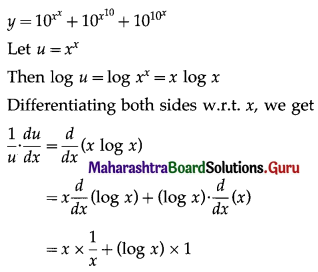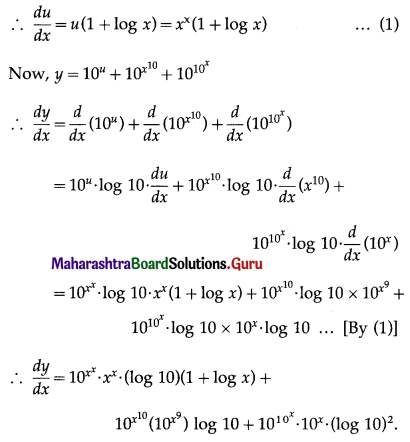# Maharashtra Board 12th Commerce Maths Solutions Chapter 3 Differentiation Ex 3.3

Balbharati Maharashtra State Board 12th Commerce Maths Solution Book Pdf Chapter 3 Differentiation Ex 3.3 Questions and Answers.

## Maharashtra State Board 12th Commerce Maths Solutions Chapter 3 Differentiation Ex 3.3

1. Find $$\frac{d y}{d x}$$ if:

Question 1.
y = $$x^{x^{2 x}}$$
Solution: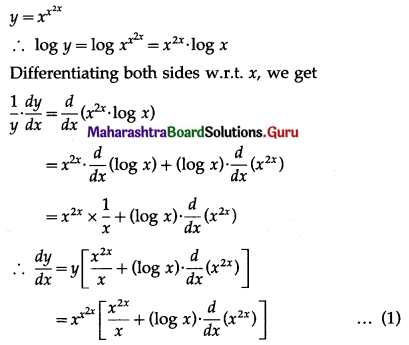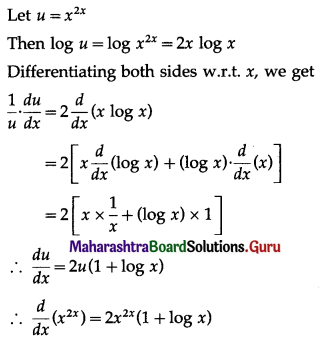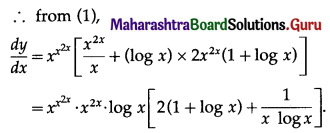Question 2.
y = $$x^{e^{x}}$$
Solution: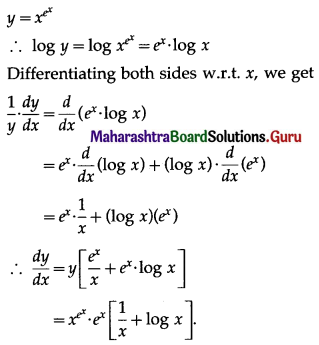Question 3.
y = $$e^{x^{x}}$$
Solution: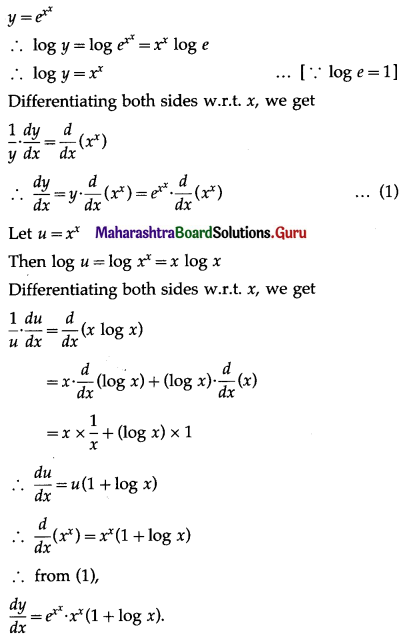2. Find $$\frac{d y}{d x}$$ if:

Question 1.
y = $$\left(1+\frac{1}{x}\right)^{x}$$
Solution: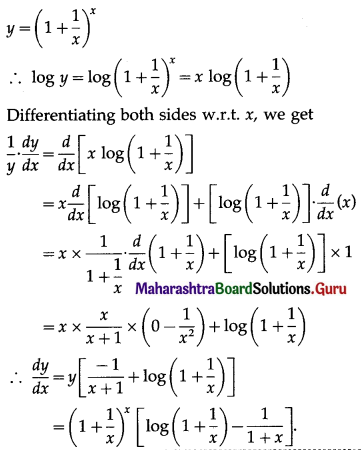Question 2.
y = (2x + 5)x
Solution: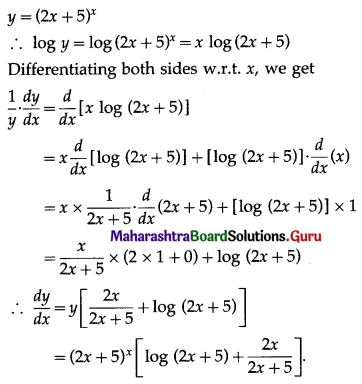Question 3.
y = $$\sqrt{\frac{(3 x-1)}{(2 x+3)(5-x)^{2}}}$$
Solution: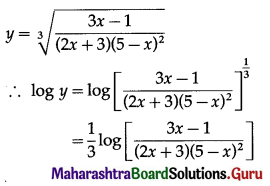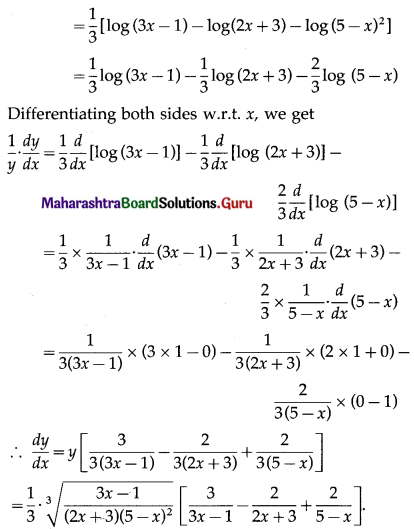3. Find $$\frac{d y}{d x}$$ if:

Question 1.
y = (log x)x + xlog x
Solution: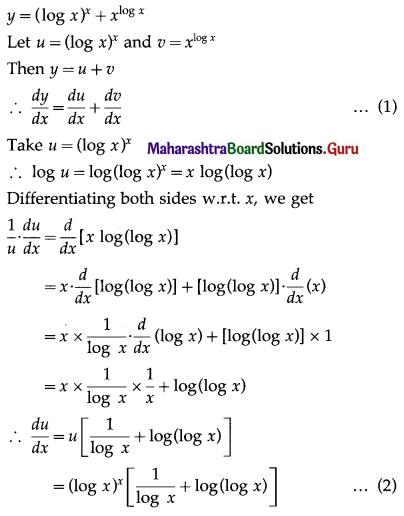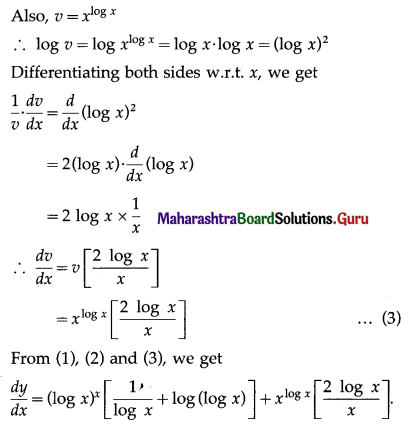Question 2.
y = xx + ax
Solution: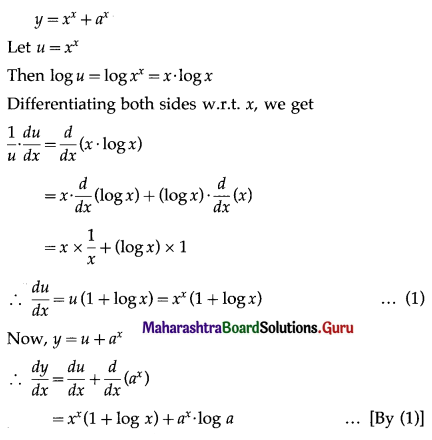Question 3.
y = $$10^{x^{x}}+10^{x^{10}}+10^{10^{x}}$$
Solution: